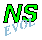## A dipole encounters a monopole

Details on the computations

There are four cases:
the monopole is weaker than each dipole half,
and the encounter is:
the monopole is nearly as strong as each dipole half,
and the encounter is:

```Initialisation
Monopole:    Bessel monopole of radius 0.5 centred at (0,0)
and strength (=circulation) :+4 or +8
==> extremum of initial vorticity:
+11.8 or +23.6
Dipole:      Lamb dipole of radius 0.75 and velocity +2
centred at (-2,0) or (-2,+0.5)
==> extrema of initial vorticity: +/- 29.5
circulation per dipole half : +/- 10.2
Tracers:     tracer contours:
- monopole: at radii 0.2 0.35 and 0.5
- dipole: at vorticity levels 0.01, 10 and 20
single tracer points:
- monopole: at the centre
- dipole: at the extrema of vorticity
Computation: domain: X = (-3, ..., 3), Y = (-3, ..., 3)
no. of grid points from wall to wall: (257, 257)
boundary conditions: stress-free along all walls
standard Arakawa scheme used
rotation effects: none
bottom topography: none
solver for streamfunction: FACR
viscosity: nu = 10^-3 (i.e. Re=1000 at T=0)
time step: dt = 0.0025
final time: T = 2 [800 time steps]
```
Contour plots:
Contours of vorticity are at 1, 2, ..., 11, 12, 15, 20 and 25, both positive and negative; there is no 0 contour.
Tracer plots:
Tracer contours are along streamlines, shown by the drawn lines; areas between the contours are filled with colours: red for the positive and bleu for the negative half of the dipole, and green for the monopole.
Note that the domain shown in the graphs on the main pages is in the vertical direction not necesarily the same as the computational domain.

Some other graphs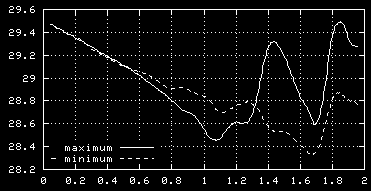The maximum and -minimum of vorticity as
function of time (averaged over 20 time steps).

The extrema of vorticity are initially of course in the two halfs of the dipole. The decrease until T=1 is due to the viscouse decay. After that the effects of the merger of the weaker monopole into the dipole set in and both vorticity extrema are affected.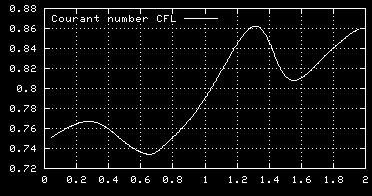The Courant number CFL, a measure for the
maximum velocity in the domain, function
of time (averaged over 20 time steps).
For the run with a monopole nearly as strong as a dipole half and an offset: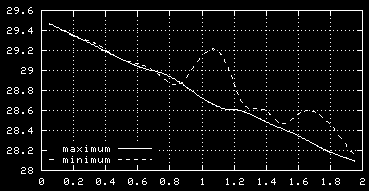The maximum and -minimum of vorticity as
function of time (averaged over 20 time steps).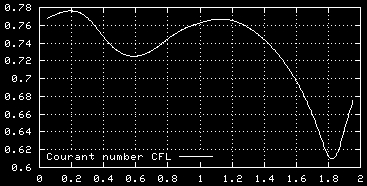The Courant number CFL, a measure for the
maximum velocity in the domain, function
of time (averaged over 20 time steps).
For the other two runs similar graphs could be made, but these suffice to give an idea.